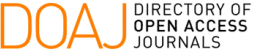### New Extension for Sub Equation Method and its Application to the Time-fractional Burgers Equation by using of Fractional Derivative

#### Abstract

In this paper, we use the new fractional complex transform and the sub equation method to study the nonlinear fractional differential equations and find the exact solutions. These solitary wave solutions demonstrate the fact that solutions to the perturbed nonlinear Schrodinger equation with power law nonlinearity model can exhibit a variety of behaviors.

PDF

#### References

S. Zhang & H.Q. Zhang. Phys. Lett. A, 375 (2011), 1069.

B. Tong, Y. He, L. Wei & X. Zhang. Phys. Lett. A, 376 (2012), 2588.

S. Guo, L. Mei, Y. Li & Y. Sun. Phys. Lett. A, 376 (2012), 407.

B. Lu. J. Math. Anal. Appl., 395 (2012), 684–693.

B. Zheng. Commun. Theor. Phys., 58 (2012), 623.

K.A. Gepreel & S. Omran. Chin. Phys. B, 21 (2012), 110–204.

G. Jumarie. Modified Riemann-Liouville derivative and fractional Taylor series of nondifferentiable functions further results. Computers and Mathematics with Applications, 51 (2006), 1367–1376.

C-s. Liu. Counter examples on Jumarie’s two basic fractional calculus formulae. Communications in Nonlinear Science and Numerical Simulation, 22(3) (2015), 9294.

I. Podlubny. Fractional Diferential Equations, Academic Press, (1999).

S.G. Samko, A.A. Kilbas & O.I. Marichev. Fractional Integrals and Derivatives: Theory and Appli- cations, Gordon and Breach Science Publishers, (1993).

A.A. Kilbas, M.H. Srivastava & J.J. Trujillo. Theory and Applications of Fractional Differential Equations, Elsevier, Amsterdam, (2006).

A.A. Kilbas & M. Saigo. On solution of integral equation of Abel-Volterra type. Diff. Integr. Equat., 8(5) (1995), 993–1011.

T. Abdeljawad. On conformable fractional calculus. Journal of Computational and Applied Mathe- matics, 279 (2015), 57–66.

T. Abdeljawad, M. Al Horani & R. Khalil. Conformable fractional semigroup operators. Journal of Semigroup Theory and Applications, (2015), Article 7, 1–9.

M. Abu Hammad & R. Khalil. Conformable heat differential equation. International Journal of Pure and Applied Mathematics, 94(2) (2014), 215–221.

R. Khalil, M. Al Horani, A. Yousef & M. Sababheh. A new definition of fractional derivative. Journal of Computational and Applied Mathematics, 264 (2014), 65–70.

DOI: https://doi.org/10.5540/tema.2017.018.02.0225

### Refbacks

• There are currently no refbacks.

Trends in Computational and Applied Mathematics

A publication of the Brazilian Society of Applied and Computational Mathematics (SBMAC)

Indexed in: